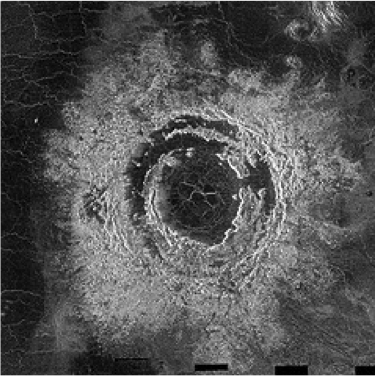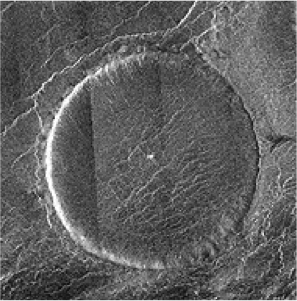# Section 10¶

In :
from IPython.display import Latex
import matplotlib.pyplot as plt
%matplotlib inline
import numpy
from matplotlib import animation, rc
from IPython.core.display import HTML
from IPython.display import HTML
from ipywidgets import *
from scipy.integrate import odeint
from IPython.display import Image


## Brief Review of Unit Conversion¶

In the homework, we've seen some consistent errors associated with converted square meters to square kilometers. Just remember that this is different than converting meters to kilometers

\begin{align} \frac{x \text{ $m^2$}}{1} &= \frac{x\text{ $m \cdot m$}}{1} \end{align}

Converting to $km^{2}$:

\begin{align} \frac{x\text{ $m\cdot m$}}{1} \cdot \frac{1\text{ km}}{1000\text{ m}}\cdot \frac{1\text{ km}}{1000\text{ m}} &= \frac{x}{1000^2}\text{km}^2 \end{align}

## Martian Surface Processes¶

#### How do we know that water flowed on the surface of Mars in the past?¶

A lot of evidence lives in and around the large craters on Mars. We see the crater rims eroded by gullies that appear to have come from running water. We see inflow channels into the craters, and outflow channels out of the craters.

Additionally, we see water valleys between lakes, and patterns on the floors of craters that are characteristic of sediment deposition.

We also see layering in rocks, indentations, and spherical shapes suggesting formation from sediment in water.

#### What evidence is there to suggest that liquid water occasionally still flows on the Martian surface?¶

With a relatively high obliquity, Mars will have seasons. This means that for part of the year the northern hemisphere is warmer, and for part of the year the southern hemisphere is warmer. In the winter, snow can accumulate on the crater walls that then melts in the summer. This melting produces gullies that change from year to year.

Furthermore, we see Recurring Slope Linea (RSL). These are features that return each summer, linear patterns striping down crater walls. These are likely from melting ground ice which finds an outlet and runs downhill. Where do you think it's most likely to find RSL's?.

#### What are the biggest differences between the southern and northern hemispheres of Mars?¶

The northern hemisphere is younger (less cratered) and lower (less elevation). This is likely due to a very large impact in the past, which left a crater the size of the northern hemisphere of Mars.

#### What other processes have been observed on Mars?¶

We've seen dust devils, global and regional dust storms, annual polar caps of CO2, permanent polar caps of water ice, polar hoods of CO2.

## Gas Escape¶

We talked briefly last week about thermal escape of gasses - the process by which quickly moving gas particles can escape the gravitational influence of the planet holding them. You'll recall that this is the process by which Hydrogen (freed from oxygen by UV dissociation) escapes the Venutian atmosphere. We'll treat this in some more detail this week.

#### Temperature and Kinetic Energy¶

Temperature and kinetic energy have a direct relationship, given by:

\begin{align} \left<KE\right> &= \frac{3}{2}k_BT\\ \left<\frac{1}{2}mv^2\right> &= \frac{3}{2}k_BT \end{align}

Where $m$ is the mass of the particle in kg, $v$ is the velocity of the particle in m/s, $k_B$ is the Boltzmann Constant ($1.38 \times 10^{-23}$ J/K), and $T$ is the temperature in Kelvin. As temperature goes up, the kinetic energy goes up at the same rate.

#### Considering the Consequences¶

Given this information, let's say we have a mixture of Nitrogen and Oxygen (like in our atmosphere) that is all at the same temperature. Which particles are moving more quickly?

Because all of the gas is at the same temperature, all of the gas has the same kinetic energy. But! Look at the equation for kinetic energy:

\begin{align} KE &= \frac{1}{2}mv^2 \end{align}

In order to keep the kinetic energy the same value, heavier particles (bigger $m$) must be moving more slowly (smaller $v$). Since Nitrogen has less mass than oxygen, it will be moving more quickly.

#### Some practice¶

What is the average speed of Hydrogen in the Sun's corona, which has a temperature in excess of 1,000,000K?

With this temperature, we can solve for the kinetic energy of the gas in the corona:

\begin{align} KE &= \frac{3}{2}k_BT\\ &= \frac{3}{2}\left(1.38 \times 10^{-23}\right)\left(1000000\right)\\ &= 2.07 \times 10^{-17} \end{align}

With the Kinetic Energy, we can solve for the velocity. Note that the mass of a Hydrogen (proton) is $1.67\times 10^{-27}$ kg.

\begin{align} KE &= \frac{1}{2}mv^2\\ v &= \sqrt{\frac{2KE}{m}} \end{align}

Substituting:

\begin{align} v &= \sqrt{\frac{2\left(2.07\times 10^{-17}\right)}{1.67\times 10^{-27}}}\\ &= 157450\text{ $m/s^2$} \end{align}

If the particle were heavier, what would happen to the velocity?

#### Escape Velocity¶

Recall that the equation for the escape velocity from a planet is given by:

\begin{align} v_{esc} &= \sqrt{\frac{2GM}{r}} \end{align}

Question: How could you derive this?

#### Reflecting on the Above¶

As the mass of a planet increases, what do you expect to observe in the rate of thermal escape of the atmosphere?

A lot of the stuff we learned in class about radar signals make more sense when you understand what radar is and how it works. It's a dead simple concept. Radar involves transmitting radio waves, having those waves bounce off of stuff, and then listening for the waves that come back to the receiver. The amount of time that it takes for the signal to return is proportional to the distance.

Think about it kind of like shining a laser pointer onto things. If you shine the laser pointer onto a rough surface, (maybe a wooden table), you're going to see some reflection back into your eyes. Why? Because it's bouncing off of a rough surface, so some of that light gets scattered back to your eyes.

Now, what if you shined that laser pointer on a mirror? Unless you had a perfect angle (which you basically never do), you're going to see no light reflected at all.

The same is true of RADAR. Rough surfaces reflect brightly, because a lot of signal gets scattered back towards the receiver. Smooth surfaces reflect most of the signal in a different direction and therefore appears dark. Slopes angled toward the receiver reflect more brightly than slopes angled away. Based on this, consider these images.

In :
PATH = "/Users/hunteradams/Documents/PhD Semester 5/Sections/"
Image(filename = PATH + "monalisa.png", width=600, height=600)

Out:In :
Image(filename = PATH + "volcano.png", width=600, height=600)

Out:In [ ]: